## Grocery

Grocery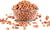Rs. 37.00
Rs. 50.00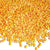Rs. 142.00
Rs. 170.00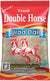Rs. 155.00
Rs. 160.00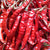Rs. 40.00
Rs. 52.00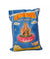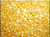Rs. 33.25
Rs. 43.00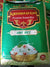Rs. 55.00
Rs. 60.00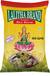Rs. 40.00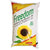Rs. 176.00
Rs. 195.00Rs. 1,300.00
Rs. 1,350.00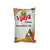Rs. 177.00
Rs. 192.00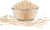Rs. 138.00
Rs. 200.00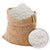Rs. 45.00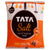Rs. 21.00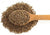Rs. 40.00
Rs. 50.00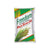Rs. 170.00
Rs. 185.00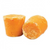Rs. 70.00
Rs. 90.00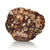Rs. 59.00
Rs. 85.00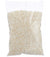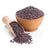Rs. 21.00
Rs. 25.00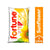Rs. 185.00
Rs. 213.00Rs. 74.00
Rs. 100.00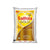Rs. 220.00
Rs. 240.00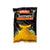Rs. 27.00
Rs. 28.00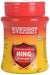Rs. 85.00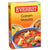Rs. 40.00
Rs. 41.00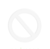Rs. 29.50
Rs. 30.00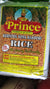Rs. 52.00
Rs. 55.00Rs. 1,300.00
Rs. 1,400.00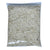Rs. 70.00
Rs. 80.00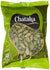Rs. 75.00
Rs. 120.00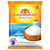Rs. 21.00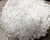Rs. 65.00
Rs. 75.00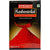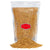Rs. 22.00
Rs. 25.00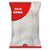Rs. 40.00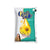Rs. 185.00
Rs. 198.00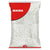Rs. 18.00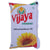Rs. 182.00
Rs. 207.00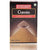Rs. 30.00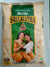Rs. 22.50
Rs. 23.00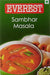Rs. 34.00Rs. 1,275.00
Rs. 1,300.00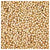Rs. 35.00
Rs. 42.00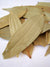Rs. 16.00
Rs. 20.00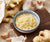Rs. 39.00
Rs. 60.00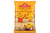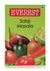Rs. 29.00
• 1